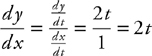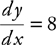# AP Calculus BC Question 374: Answer and Explanation

### Test Information

Question: 374

4. Which of the following is an equation of the tangent line to the curve described by the parametric equations x = t and y = t2 when t = 4 ?

• A. y = 8
• B. y = 2x + 8
• C. y = 8x - 16
• D. y = 8x + 16

When t = 4, you have x = 4 and y = 16, and thus the point (4, 16) is on the curve. The slope of the tangent line isand when t = 4,. The equation of the tangent line is y - 16 = 8(x - 4) or y = 8x - 16.